• Slides: 31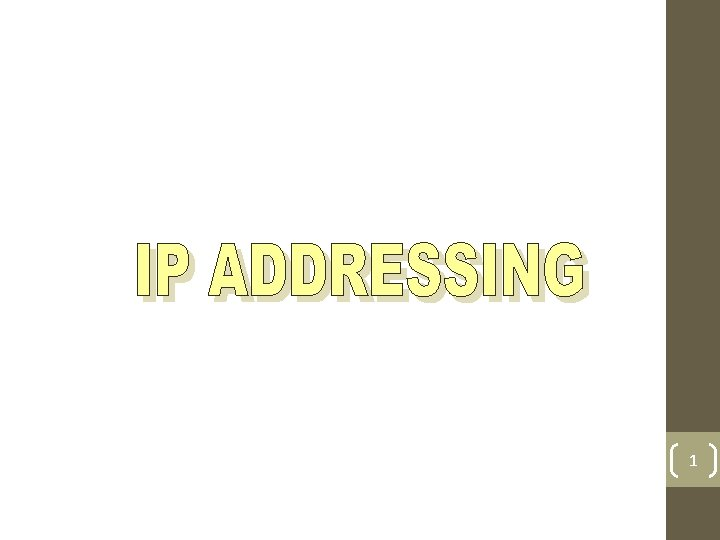1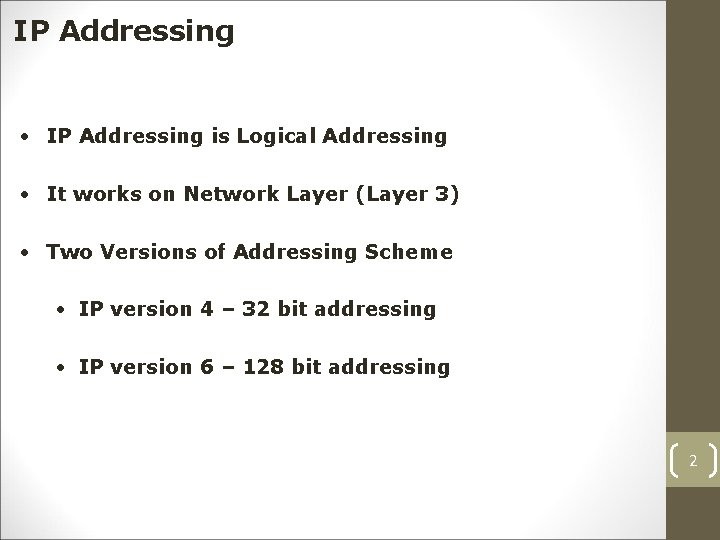IP Addressing • IP Addressing is Logical Addressing • It works on Network Layer (Layer 3) • Two Versions of Addressing Scheme • IP version 4 – 32 bit addressing • IP version 6 – 128 bit addressing 2IP version 4 Bit is a value that will represent 0’s or 1’s (i. e. Binary) 0101000001011011111100000001 • 32 bits are divided into 4 Octets known as Dotted Decimal Notation First Octet Second Octet Third Octet Forth Octet 0101. 00000101. 10111111. 00000001 3IP version 6 • 128 -bit address is divided along 16 -bit boundaries, and each 16 -bit block is converted to a 4 -digit hexadecimal number and separated by colons (Colon -Hex Notation) FEDC: BA 98: 7654: 3210: FEDC: BA 98: 7654: 3210 4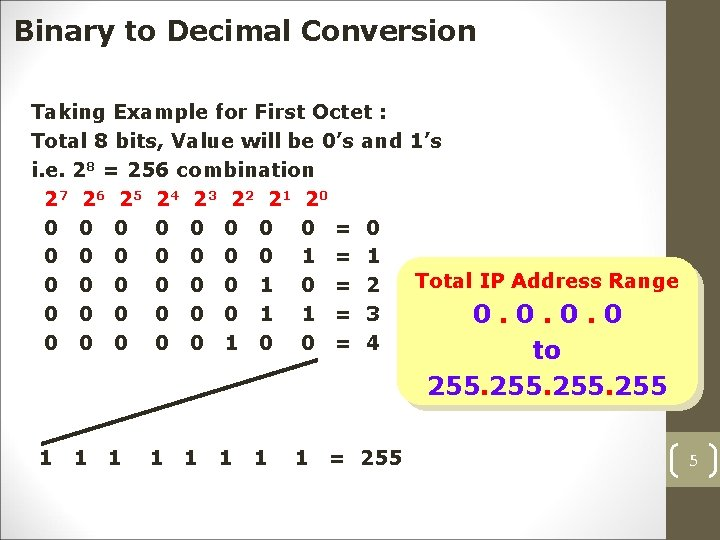Binary to Decimal Conversion Taking Example for First Octet : Total 8 bits, Value will be 0’s and 1’s i. e. 28 = 256 combination 27 26 25 24 23 22 21 20 0 0 0 0 = 0 0 0 0 1 = 1 Total IP Address Range 0 0 0 1 0 = 2 0 0 0 1 1 = 3 0. 0 0 0 1 0 0 = 4 to 255 1 1 1 1 = 255 5IP Address Classes • Total IP Addressing Scheme is divided into 5 Classes • CLASS A • CLASS B LAN & WAN • CLASS C • CLASS D Multicasting • CLASS E Research & Development 6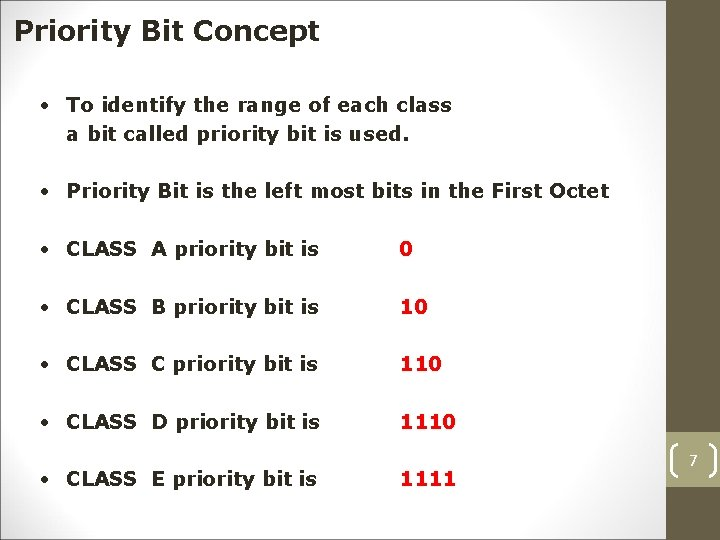Priority Bit Concept • To identify the range of each class a bit called priority bit is used. • Priority Bit is the left most bits in the First Octet • CLASS A priority bit is 0 • CLASS B priority bit is 10 • CLASS C priority bit is 110 • CLASS D priority bit is 1110 • CLASS E priority bit is 1111 7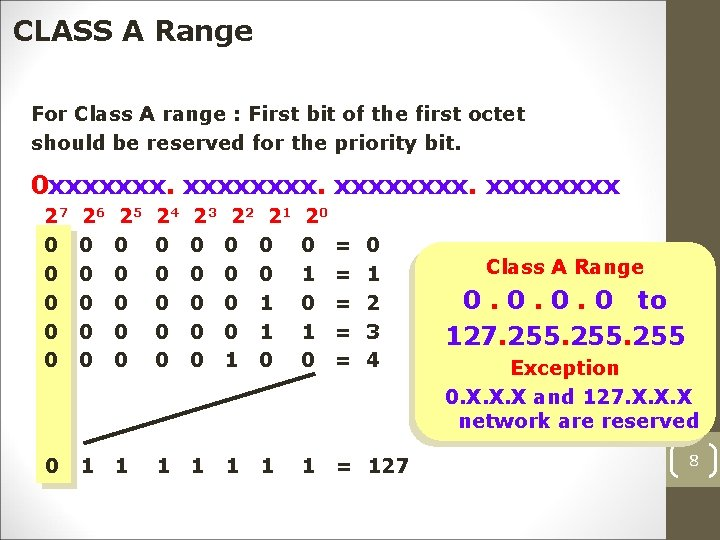CLASS A Range For Class A range : First bit of the first octet should be reserved for the priority bit. 0 xxxxxxxx. xxxx 27 0 0 0 26 0 0 0 25 0 0 0 24 0 0 0 23 0 0 0 22 21 0 0 0 1 1 0 20 0 1 0 = = = 0 1 1 1 = 127 1 0 1 2 3 4 Class A Range 0. 0 to 127. 255 Exception 0. X. X. X and 127. X. X. X network are reserved 8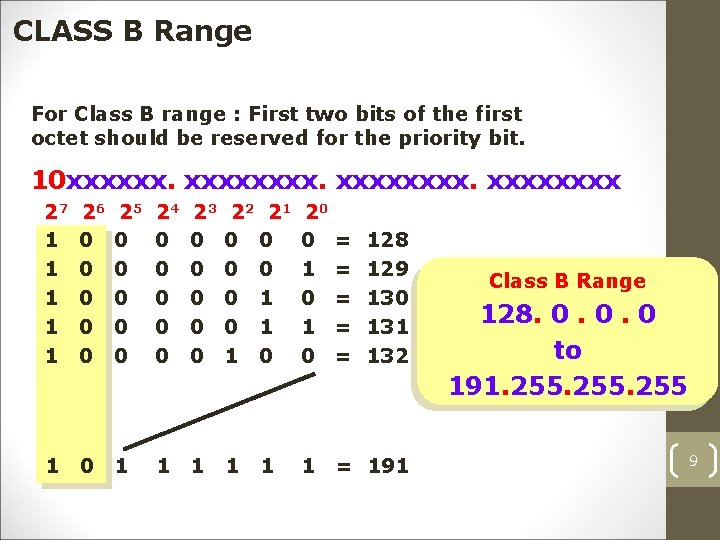CLASS B Range For Class B range : First two bits of the first octet should be reserved for the priority bit. 10 xxxxxxxx. xxxx 27 1 1 1 26 0 0 0 25 0 0 0 24 0 0 0 23 0 0 0 22 21 0 0 0 1 1 0 20 0 1 0 = = = 1 0 1 1 1 = 191 1 128 129 130 131 132 Class B Range 128. 0. 0. 0 to 191. 255 9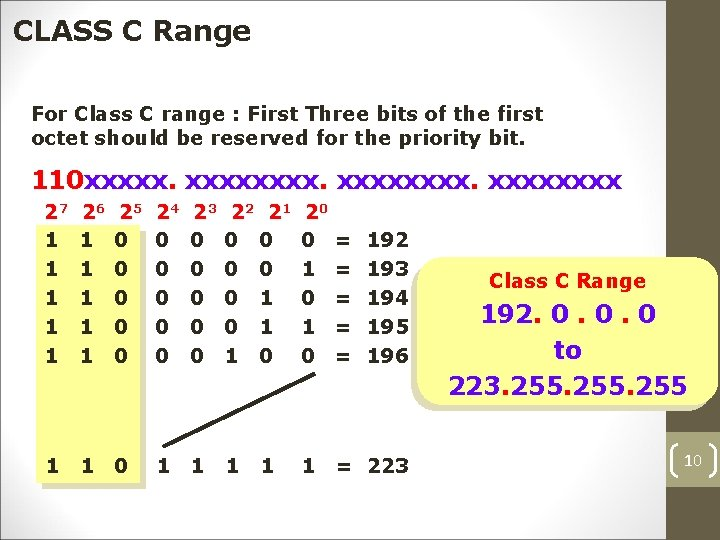CLASS C Range For Class C range : First Three bits of the first octet should be reserved for the priority bit. 110 xxxxxxxx. xxxx 27 1 1 1 26 1 1 1 25 0 0 0 24 0 0 0 23 0 0 0 22 21 0 0 0 1 1 0 20 0 1 0 = = = 1 1 0 1 1 = 223 1 192 193 194 195 196 Class C Range 192. 0. 0. 0 to 223. 255 10CLASS D Range For Class D range : First four bits of the first octet should be reserved for the priority bit. 1110 xxxxxxxx 27 1 1 1 26 1 1 1 25 1 1 1 24 0 0 0 23 0 0 0 22 21 0 0 0 1 1 0 20 0 1 0 = = = 1 1 1 0 1 1 1 = 239 1 224 225 226 227 228 Class D Range 224. 0. 0. 0 to 239. 255 11CLASS E Range For Class E range : First four bits of the first octet should be reserved for the priority bit. 1111 xxxxxxxx 27 1 1 1 26 1 1 1 25 1 1 1 24 1 1 1 23 0 0 0 22 21 0 0 0 1 1 1 1 20 0 1 0 1 = = = 240 241 242 243 244 = 255 Class E Range 240. 0 to 255 12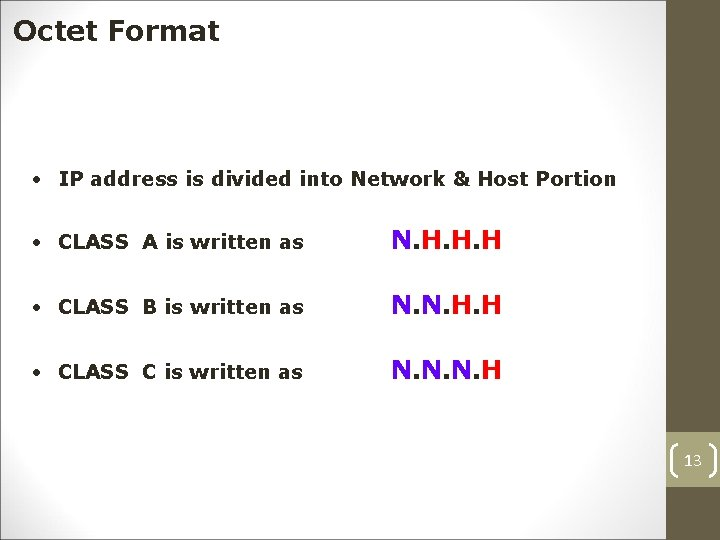Octet Format • IP address is divided into Network & Host Portion • CLASS A is written as N. H. H. H • CLASS B is written as N. N. H. H • CLASS C is written as N. N. N. H 13CLASS A – Networks & Host CLASS A • Class A Octet Format is N. H. H. H • Network bits : 8 • No. = = = 128 Networks & 16777214 Hosts/NW Host bits : 24 of Networks 28 27 128 of Host 224 – 2 (-2 is for Network ID & Broadcast ID) 16777216 - 2 16777214 Hosts/Network 14CLASS B – Networks & Host CLASS B • Class B Octet Format is N. N. H. H • Network bits : 16 16384 Networks & 65534 Hosts/NW Host bits : 16 • No. of Networks = 216 = 16384 Networks • No. = = = of Host 216 – 2 (-2 is for Network ID & Broadcast ID) 65536 - 2 65534 Hosts/Network 15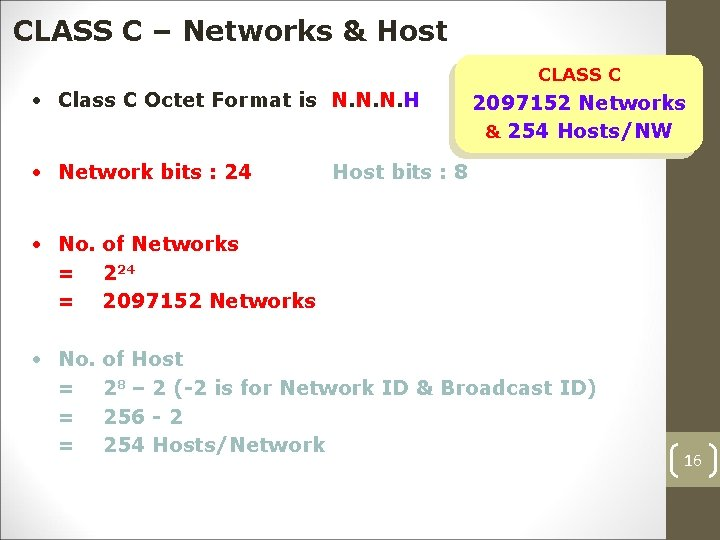CLASS C – Networks & Host CLASS C • Class C Octet Format is N. N. N. H • Network bits : 24 2097152 Networks & 254 Hosts/NW Host bits : 8 • No. of Networks = 224 = 2097152 Networks • No. = = = of Host 28 – 2 (-2 is for Network ID & Broadcast ID) 256 - 2 254 Hosts/Network 16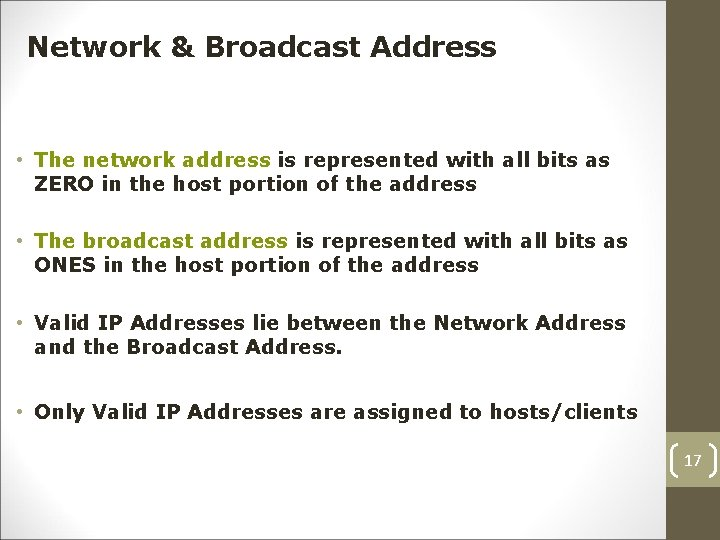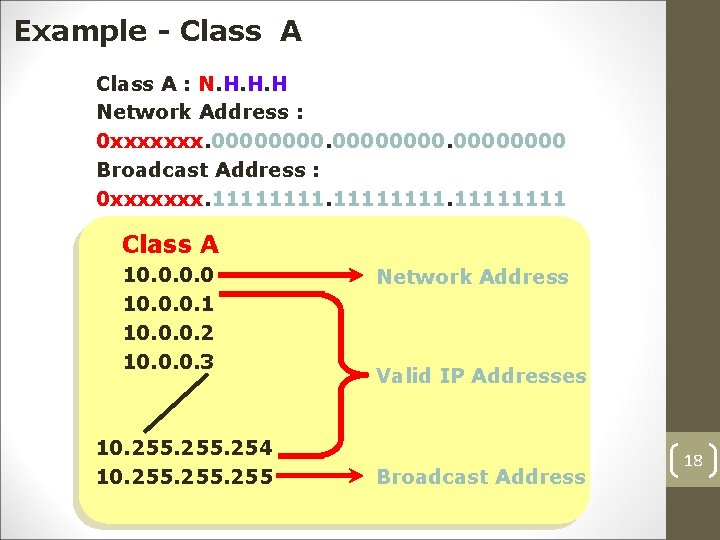Example - Class A : N. H. H. H Network Address : 0 xxxxxxx. 00000000 Broadcast Address : 0 xxxxxxx. 11111111 Class A 10. 0. 0. 1 10. 0. 0. 2 10. 0. 0. 3 10. 255. 254 10. 255 Network Address Valid IP Addresses Broadcast Address 18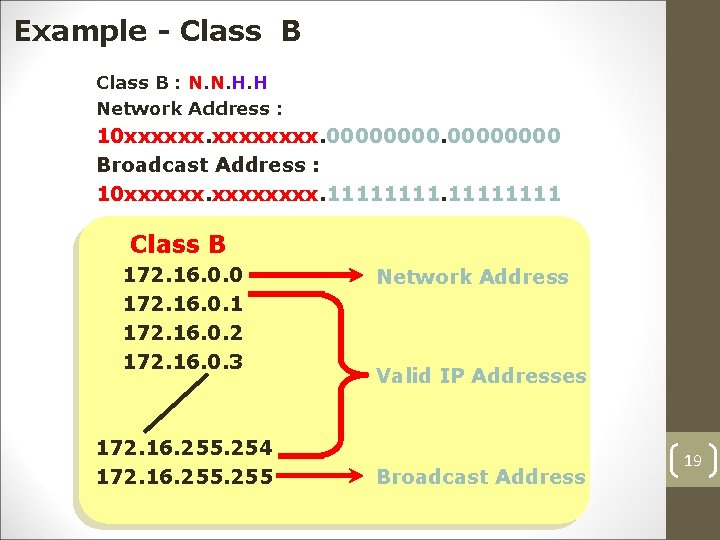Example - Class B : N. N. H. H Network Address : 10 xxxxxx. 00000000 Broadcast Address : 10 xxxxxx. 11111111 Class B 172. 16. 0. 0 172. 16. 0. 1 172. 16. 0. 2 172. 16. 0. 3 172. 16. 255. 254 172. 16. 255 Network Address Valid IP Addresses Broadcast Address 19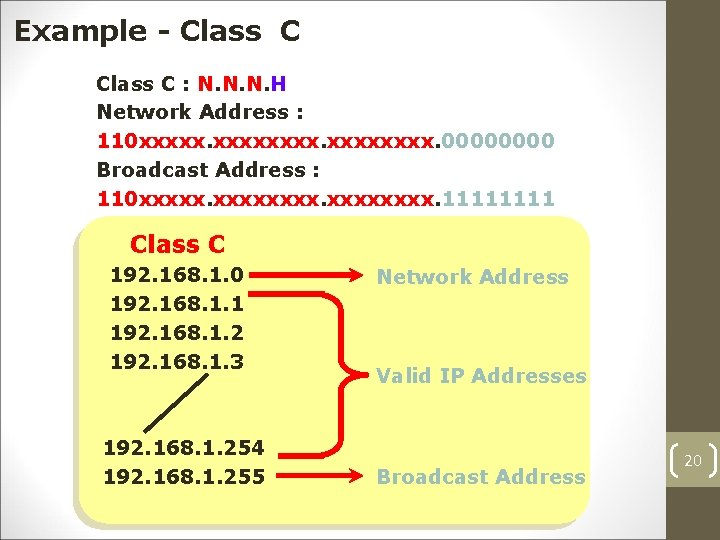Example - Class C : N. N. N. H Network Address : 110 xxxxxxxx. 0000 Broadcast Address : 110 xxxxxxxx. 1111 Class C 192. 168. 1. 0 192. 168. 1. 1 192. 168. 1. 2 192. 168. 1. 3 192. 168. 1. 254 192. 168. 1. 255 Network Address Valid IP Addresses Broadcast Address 20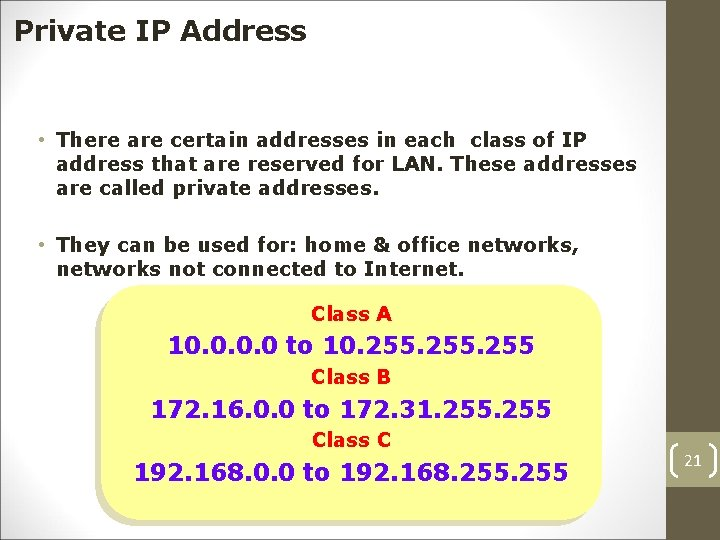Private IP Address • There are certain addresses in each class of IP address that are reserved for LAN. These addresses are called private addresses. • They can be used for: home & office networks, networks not connected to Internet. Class A 10. 0 to 10. 255 Class B 172. 16. 0. 0 to 172. 31. 255 Class C 192. 168. 0. 0 to 192. 168. 255 21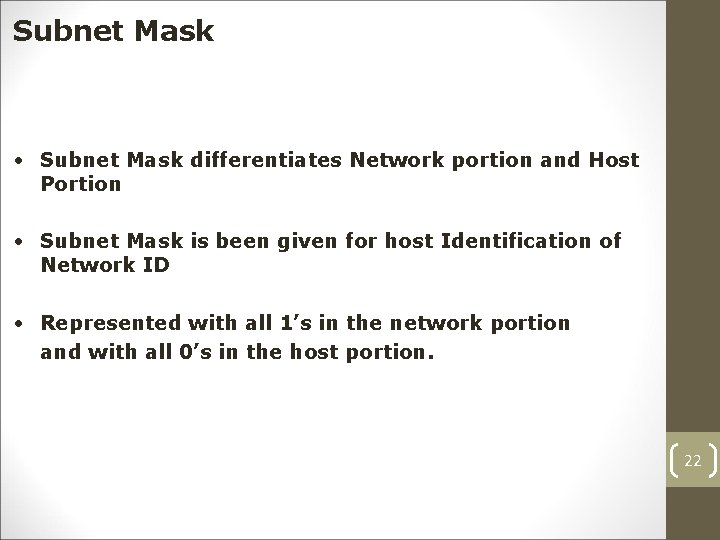Subnet Mask • Subnet Mask differentiates Network portion and Host Portion • Subnet Mask is been given for host Identification of Network ID • Represented with all 1’s in the network portion and with all 0’s in the host portion. 22Subnet Mask - Examples Class A : N. H. H. H 1111. 00000000 Default Subnet Mask for Class A is 255. 0. 0. 0 Class B : N. N. H. H 11111111. 0000 Default Subnet Mask for Class B is 255. 0. 0 Class C : N. N. N. H 11111111. 0000 Default Subnet Mask for Class C is 255. 0 23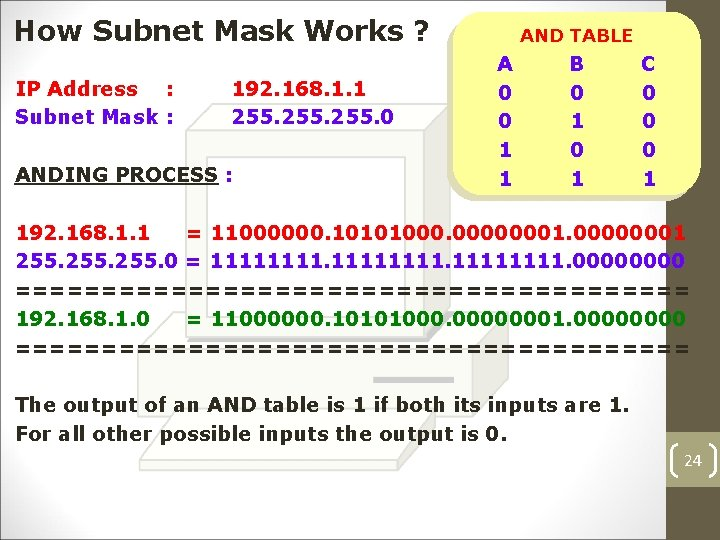How Subnet Mask Works ? IP Address : Subnet Mask : 192. 168. 1. 1 255. 0 ANDING PROCESS : AND TABLE A 0 0 1 1 B 0 1 C 0 0 0 1 192. 168. 1. 1 = 11000000. 10101000. 00000001 255. 0 = 11111111. 0000 ==================== 192. 168. 1. 0 = 11000000. 10101000. 00000001. 0000 ==================== The output of an AND table is 1 if both its inputs are 1. For all other possible inputs the output is 0. 24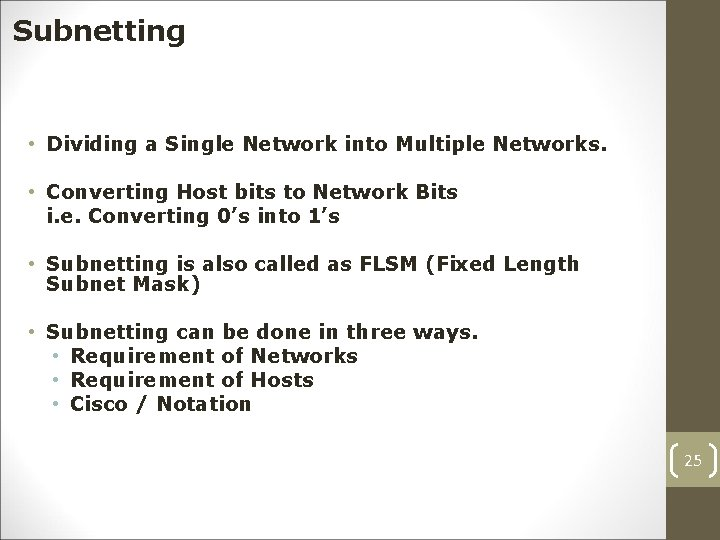Subnetting • Dividing a Single Network into Multiple Networks. • Converting Host bits to Network Bits i. e. Converting 0’s into 1’s • Subnetting is also called as FLSM (Fixed Length Subnet Mask) • Subnetting can be done in three ways. • Requirement of Networks • Requirement of Hosts • Cisco / Notation 25Scenario Suppose, In Jazan University we have 100 PC • Which Class is preferred for the network ? Answer : Class C. • We have Five Buildings with 20 Pcs each Jazan University – 192. 168. 1. 0/24 – – – Dean Bldg Admin Bldg 07 Bldg 08 Bldg 13 192. 168. 1. 1 192. 168. 1. 21 192. 168. 1. 41 192. 168. 1. 61 192. 168. 1. 81 to 192. 168. 1. 20 to 192. 168. 1. 40 to 192. 168. 1. 60 to 192. 168. 1. 80 to 192. 168. 1. 100 26Power table 21 = 2 29 = 512 217 = 131072 225 = 33554432 22 = 4 210 = 1024 218 = 262144 226 = 67108864 23 = 8 211 = 2048 219 = 524288 227 = 134217728 24 = 16 212 = 4096 220 = 1048576 228 = 268435456 25 = 32 213 = 8192 221 = 2097152 229 = 536870912 26 = 64 214 = 16384 222 = 4194304 230 = 1073741824 27 = 128 215 = 32768 223 = 8388608 231 = 2147483648 28 232 = 256 216 = 65536 224 = 16777216 27 = 4294967296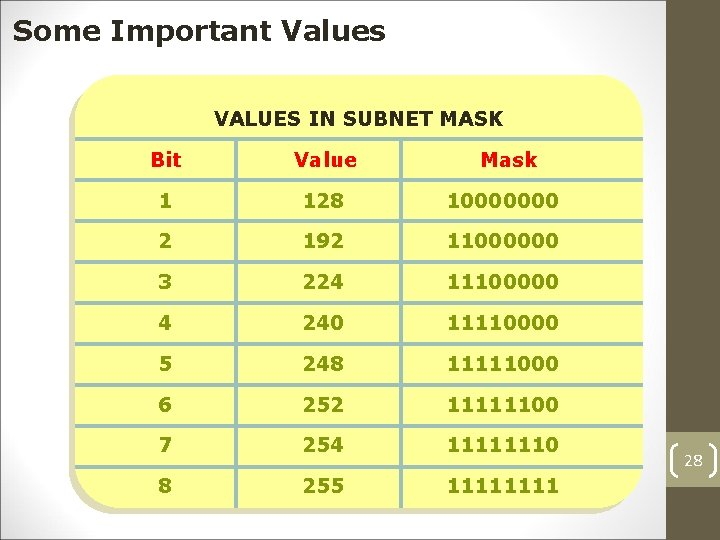Some Important Values VALUES IN SUBNET MASK Bit Value Mask 1 128 10000000 2 192 11000000 3 224 11100000 4 240 11110000 5 248 11111000 6 252 11111100 7 254 11111110 8 255 1111 28Requirement of Networks is 5 ? Example – 1 Class C : N. N. N. H 110 xxxxxxxx. xxxx Class C : 192. 168. 1. 0 • No. = = of Subnet 2 n – 2 Req. of Subnet 23 – 2 5 (-2 is for First & Last Subnet Range) 8– 2 6 Subnet • No. = = of Host 2 h – 2 (-2 is for Network ID & Broadcast ID) 25 – 2 32 – 2 30 Hosts/Subnet 29 HELPExample – 1 (Continued…) • Customize Subnet Mask = 255. 224 1 2 8 6 4 3 2 11111111. 11100000 • Range of Networks Network ID 192. 168. 1. 0 192. 168. 1. 32 192. 168. 1. 64 192. 168. 1. 96 192. 168. 1. 128 192. 168. 1. 160 192. 168. 1. 224 Broadcast ID 192. 168. 1. 31 x 192. 168. 1. 63 192. 168. 1. 95 192. 168. 1. 127 Valid Subnets 192. 168. 1. 159 192. 168. 1. 191 30 192. 168. 1. 223 192. 168. 1. 255 x31